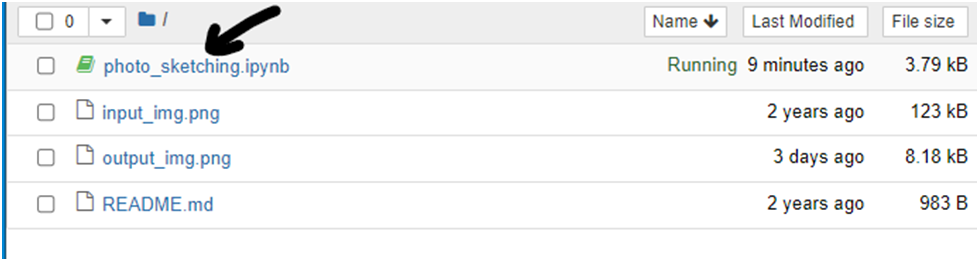TechieYan Technologies

# Convert Image To A Pencil Sketch Using Python Project

## Abstract

In Python, an image is just a two-dimensional array of integers. So one can do a couple of matrix manipulations using various python modules in order to get some very interesting effects. In order to convert the normal image to a sketch, we will change the original image of RGB values to gray scale image, in this way a sketch of the input image will be generated. There are processes in converting image into pencil sketch from duplicating layer to applying Gaussian blur filter all the steps are executed by the means of programming in Python language. This project will be fast, efficient and very useful to perform conversion. A small code that can convert an image to a pencil sketch. An image is basically an array of numbers to Python. So we can perform a variety of matrix manipulations to get some very interesting results. This code shows how to transform an image into a ‘pencil’ outline. The whole procedure is done by taking sample kernel/filter and this filter is applied to the entire image which is in the form of array. As you change the filter size and elements in it you can gradually see the change in the image.Programming Methodology

• The following steps to be followed :-

1. Read the input image as gray scale image
1. Remove any noise using a gaussian filter
1. Create a negative image (invert the image)
1. Dodge these two images (gray and inverted images). These two images reinforces some edges and complements some edges. Alternatively, these two images can be blended with different weights
1. Show the image and wait for a keystroke to close the display

## How to Execute?

Step 1 :

You need to open the jupyter notebook in the path directory on the anaconda prompt shell.

In my caseTo perform the above step you already need to have knowledge of installing jupyter notebook and running anaconda shell, if you don’t know how to install or run jupyter notebook check our previous articles about it.

Step 2 :

Open   .ipynb file from the folderStep 3 :

Install required libraries using command pip install (library_name) for example

pip install open-cv python

necessary libraries are ( open-cv python , matplotlib , numpy )

these libraries should be installed in an empty cell , to get it click on + symbol on the notebook.

Step 4 :

Now you need to run each cell individually by run command or by using shortcut key shift+enter

In the 3 and 4 cell you will see the original image like this